# Triangulation survey

6. Apr 2018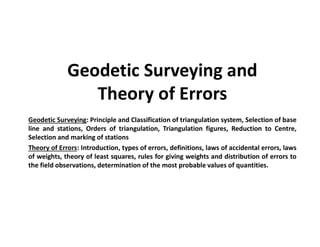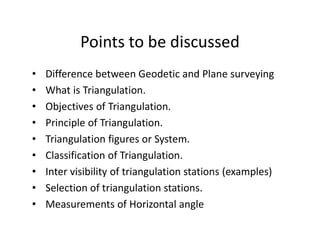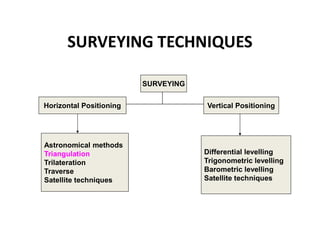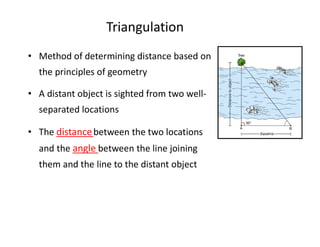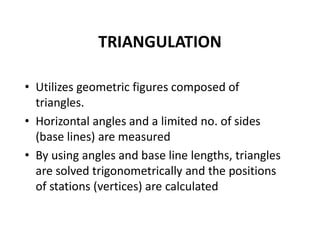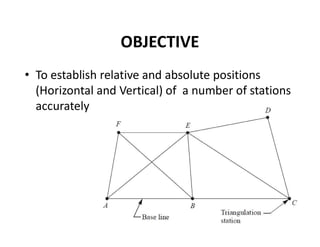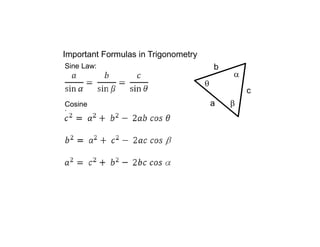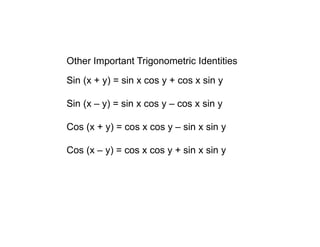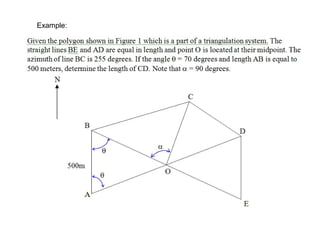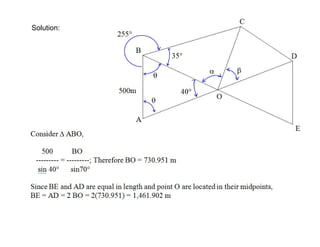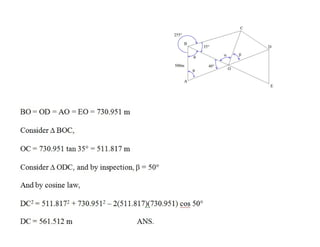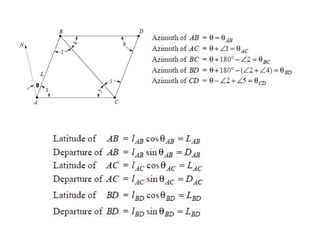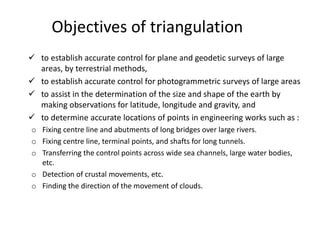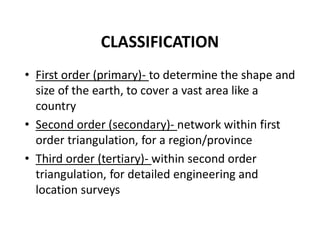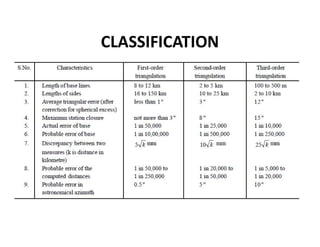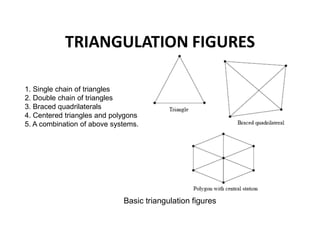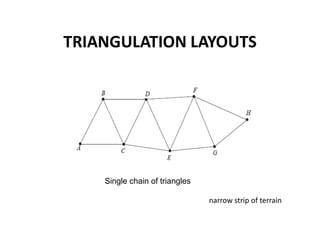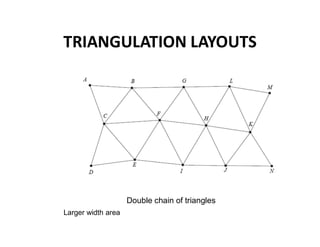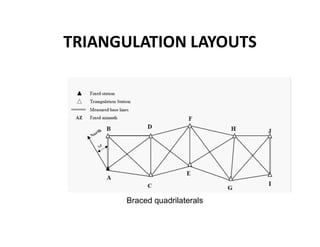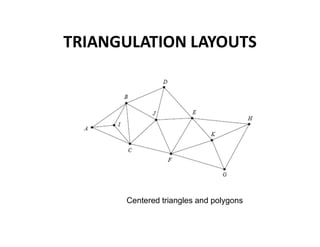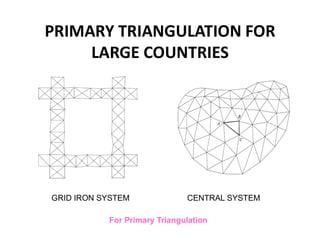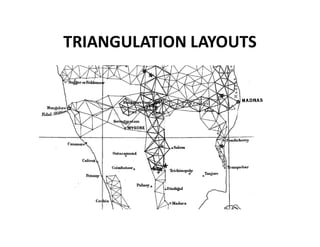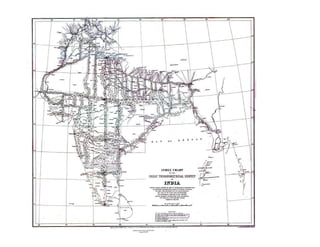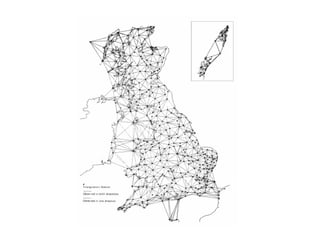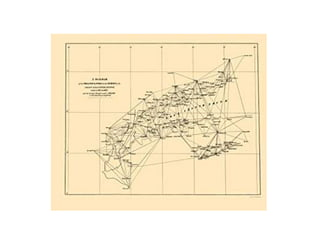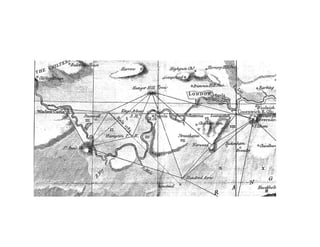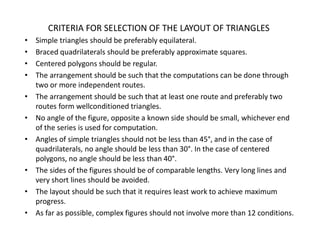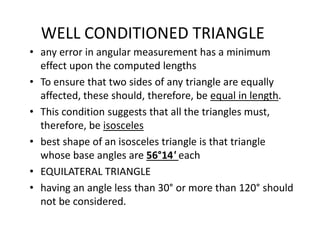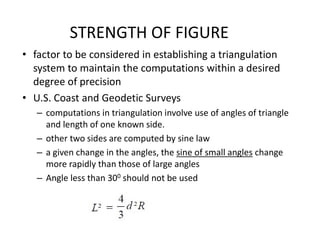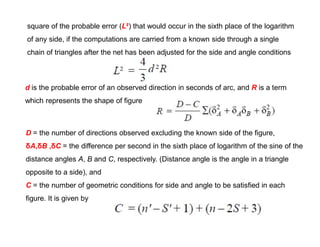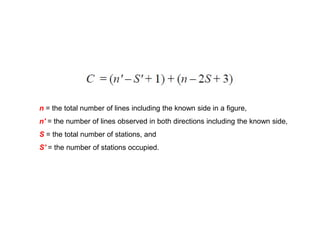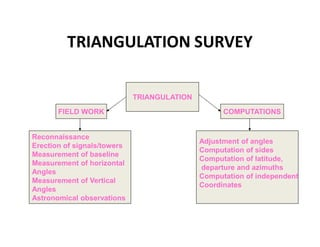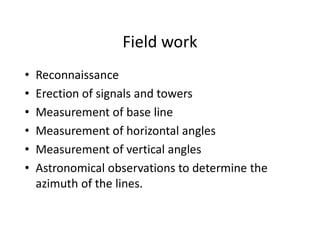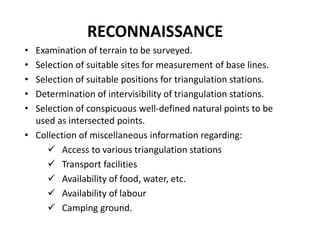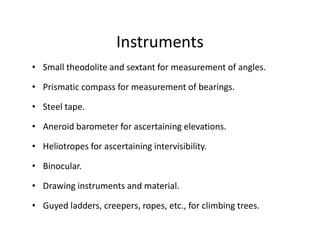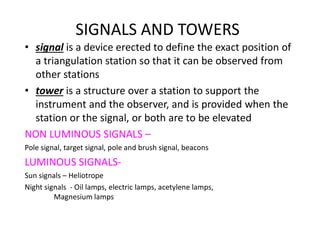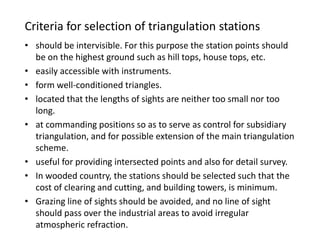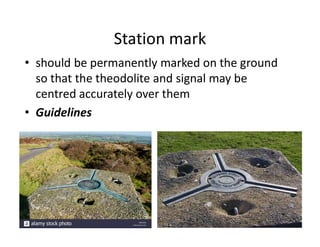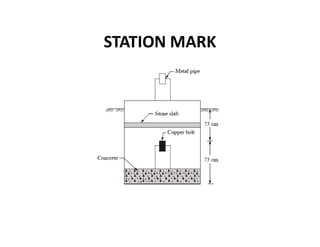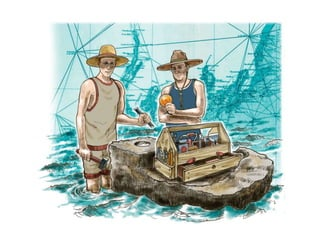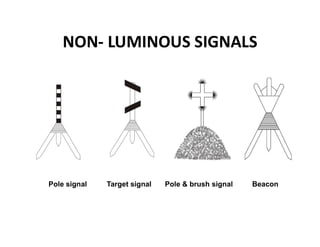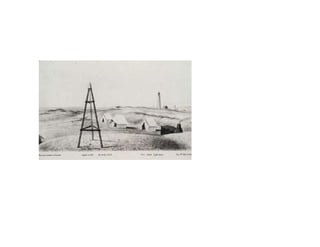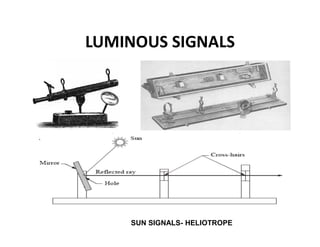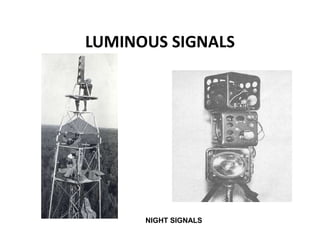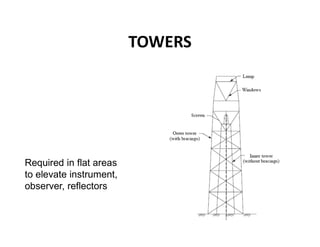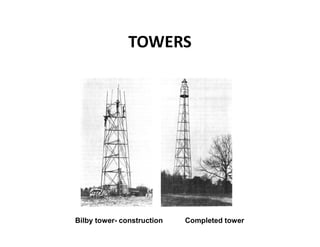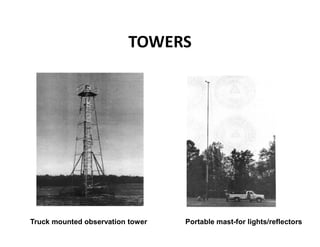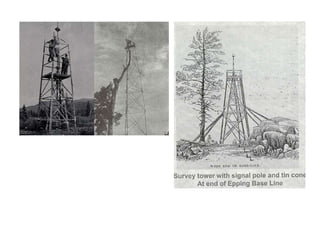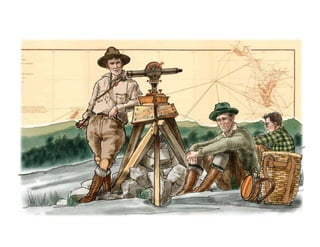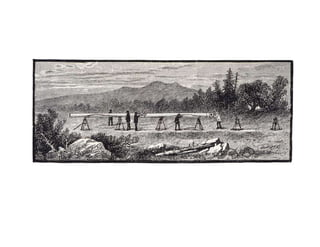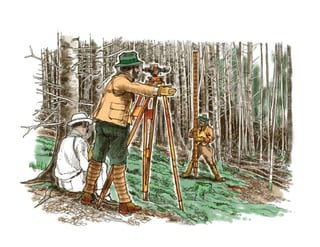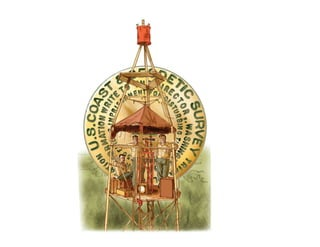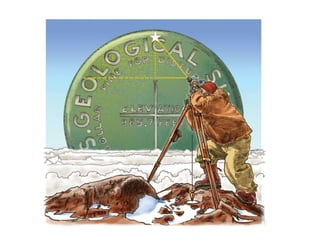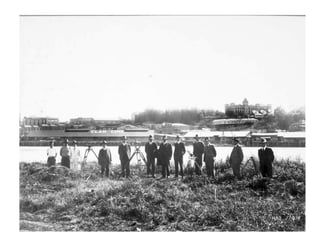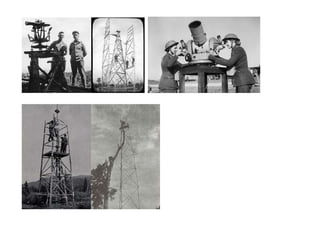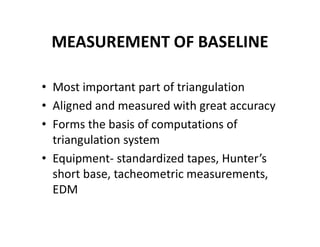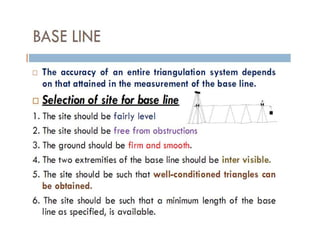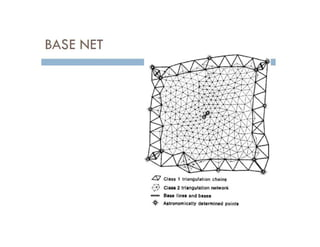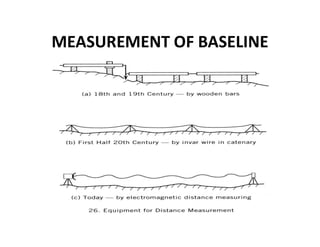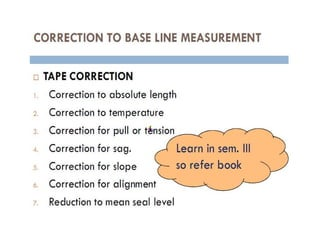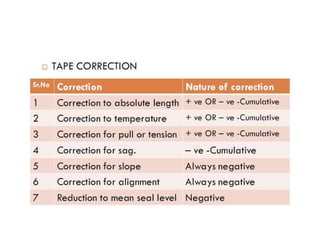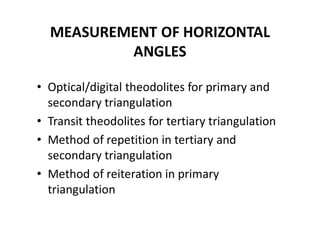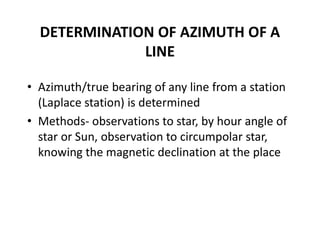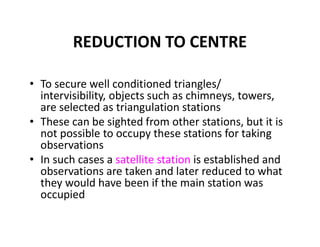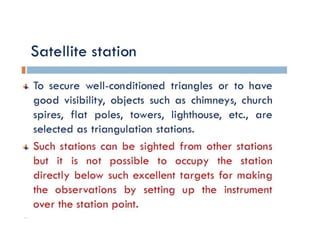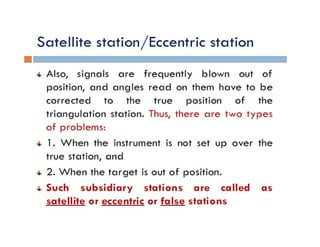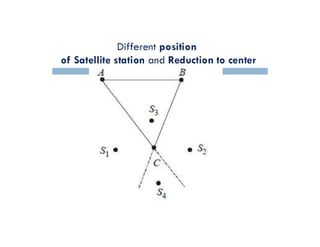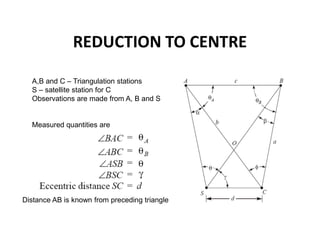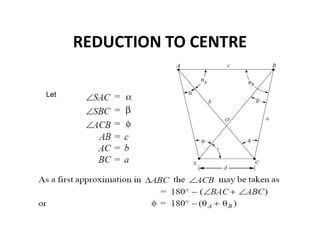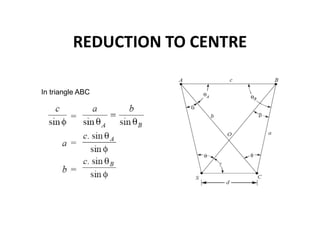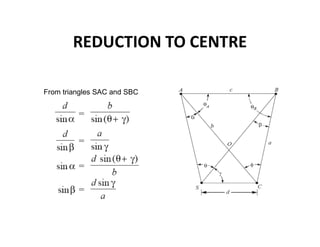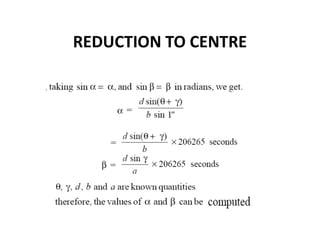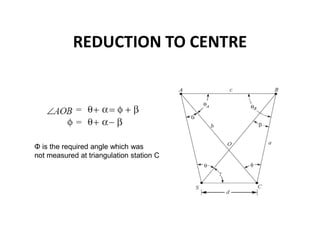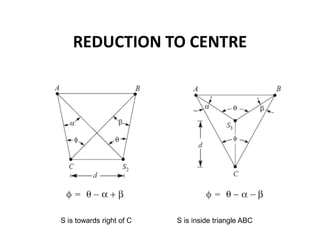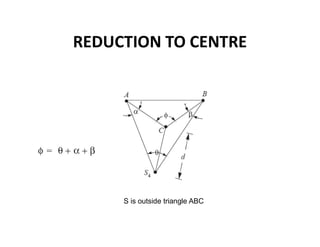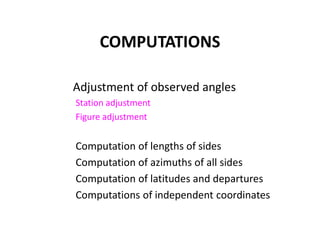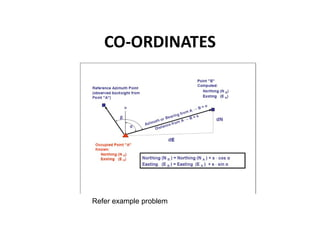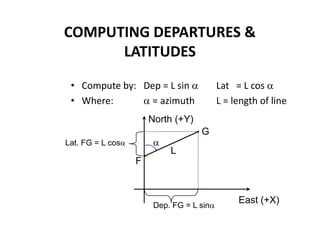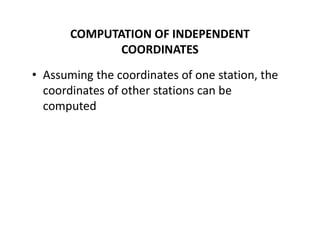1 von 79

### Triangulation survey

• 1. Geodetic Surveying and Theory of Errors Geodetic Surveying: Principle and Classification of triangulation system, Selection of base line and stations, Orders of triangulation, Triangulation figures, Reduction to Centre, Selection and marking of stations Theory of Errors: Introduction, types of errors, definitions, laws of accidental errors, laws of weights, theory of least squares, rules for giving weights and distribution of errors to the field observations, determination of the most probable values of quantities.
• 2. Points to be discussed • Difference between Geodetic and Plane surveying • What is Triangulation. • Objectives of Triangulation. • Principle of Triangulation. • Triangulation figures or System. • Classification of Triangulation. • Inter visibility of triangulation stations (examples) • Selection of triangulation stations. • Measurements of Horizontal angle
• 3. SURVEYING TECHNIQUES SURVEYING Horizontal Positioning Vertical Positioning Astronomical methods Triangulation Trilateration Traverse Satellite techniques Differential levelling Trigonometric levelling Barometric levelling Satellite techniques
• 4. Triangulation • Method of determining distance based on the principles of geometry • A distant object is sighted from two well- separated locations • The distancebetween the two locations and the angle between the line joining them and the line to the distant object
• 5. TRIANGULATION • Utilizes geometric figures composed of triangles. • Horizontal angles and a limited no. of sides (base lines) are measured • By using angles and base line lengths, triangles are solved trigonometrically and the positions of stations (vertices) are calculated
• 6. OBJECTIVE • To establish relative and absolute positions (Horizontal and Vertical) of a number of stations accurately
• 7. Important Formulas in Trigonometry    c b a Sine Law: Cosine Law:
• 8. Other Important Trigonometric Identities Sin (x + y) = sin x cos y + cos x sin y Sin (x – y) = sin x cos y – cos x sin y Cos (x + y) = cos x cos y – sin x sin y Cos (x – y) = cos x cos y + sin x sin y
• 13. Objectives of triangulation  to establish accurate control for plane and geodetic surveys of large areas, by terrestrial methods,  to establish accurate control for photogrammetric surveys of large areas  to assist in the determination of the size and shape of the earth by making observations for latitude, longitude and gravity, and  to determine accurate locations of points in engineering works such as : o Fixing centre line and abutments of long bridges over large rivers. o Fixing centre line, terminal points, and shafts for long tunnels. o Transferring the control points across wide sea channels, large water bodies, etc. o Detection of crustal movements, etc. o Finding the direction of the movement of clouds.
• 14. CLASSIFICATION • First order (primary)- to determine the shape and size of the earth, to cover a vast area like a country • Second order (secondary)- network within first order triangulation, for a region/province • Third order (tertiary)- within second order triangulation, for detailed engineering and location surveys
• 16. TRIANGULATION FIGURES Basic triangulation figures 1. Single chain of triangles 2. Double chain of triangles 3. Braced quadrilaterals 4. Centered triangles and polygons 5. A combination of above systems.
• 17. TRIANGULATION LAYOUTS Single chain of triangles narrow strip of terrain
• 18. TRIANGULATION LAYOUTS Double chain of triangles Larger width area
• 21. PRIMARY TRIANGULATION FOR LARGE COUNTRIES GRID IRON SYSTEM CENTRAL SYSTEM For Primary Triangulation
• 27. CRITERIA FOR SELECTION OF THE LAYOUT OF TRIANGLES • Simple triangles should be preferably equilateral. • Braced quadrilaterals should be preferably approximate squares. • Centered polygons should be regular. • The arrangement should be such that the computations can be done through two or more independent routes. • The arrangement should be such that at least one route and preferably two routes form wellconditioned triangles. • No angle of the figure, opposite a known side should be small, whichever end of the series is used for computation. • Angles of simple triangles should not be less than 45°, and in the case of quadrilaterals, no angle should be less than 30°. In the case of centered polygons, no angle should be less than 40°. • The sides of the figures should be of comparable lengths. Very long lines and very short lines should be avoided. • The layout should be such that it requires least work to achieve maximum progress. • As far as possible, complex figures should not involve more than 12 conditions.
• 28. WELL CONDITIONED TRIANGLE • any error in angular measurement has a minimum effect upon the computed lengths • To ensure that two sides of any triangle are equally affected, these should, therefore, be equal in length. • This condition suggests that all the triangles must, therefore, be isosceles • best shape of an isosceles triangle is that triangle whose base angles are 56°14' each • EQUILATERAL TRIANGLE • having an angle less than 30° or more than 120° should not be considered.
• 29. STRENGTH OF FIGURE • factor to be considered in establishing a triangulation system to maintain the computations within a desired degree of precision • U.S. Coast and Geodetic Surveys – computations in triangulation involve use of angles of triangle and length of one known side. – other two sides are computed by sine law – a given change in the angles, the sine of small angles change more rapidly than those of large angles – Angle less than 300 should not be used
• 30. square of the probable error (L²) that would occur in the sixth place of the logarithm of any side, if the computations are carried from a known side through a single chain of triangles after the net has been adjusted for the side and angle conditions d is the probable error of an observed direction in seconds of arc, and R is a term which represents the shape of figure D = the number of directions observed excluding the known side of the figure, δA,δB ,δC = the difference per second in the sixth place of logarithm of the sine of the distance angles A, B and C, respectively. (Distance angle is the angle in a triangle opposite to a side), and C = the number of geometric conditions for side and angle to be satisfied in each figure. It is given by
• 31. n = the total number of lines including the known side in a figure, n' = the number of lines observed in both directions including the known side, S = the total number of stations, and S' = the number of stations occupied.
• 32. TRIANGULATION SURVEY TRIANGULATION FIELD WORK COMPUTATIONS Reconnaissance Erection of signals/towers Measurement of baseline Measurement of horizontal Angles Measurement of Vertical Angles Astronomical observations Adjustment of angles Computation of sides Computation of latitude, departure and azimuths Computation of independent Coordinates
• 33. Field work • Reconnaissance • Erection of signals and towers • Measurement of base line • Measurement of horizontal angles • Measurement of vertical angles • Astronomical observations to determine the azimuth of the lines.
• 34. RECONNAISSANCE • Examination of terrain to be surveyed. • Selection of suitable sites for measurement of base lines. • Selection of suitable positions for triangulation stations. • Determination of intervisibility of triangulation stations. • Selection of conspicuous well-defined natural points to be used as intersected points. • Collection of miscellaneous information regarding:  Access to various triangulation stations  Transport facilities  Availability of food, water, etc.  Availability of labour  Camping ground.
• 35. Instruments • Small theodolite and sextant for measurement of angles. • Prismatic compass for measurement of bearings. • Steel tape. • Aneroid barometer for ascertaining elevations. • Heliotropes for ascertaining intervisibility. • Binocular. • Drawing instruments and material. • Guyed ladders, creepers, ropes, etc., for climbing trees.
• 36. SIGNALS AND TOWERS • signal is a device erected to define the exact position of a triangulation station so that it can be observed from other stations • tower is a structure over a station to support the instrument and the observer, and is provided when the station or the signal, or both are to be elevated NON LUMINOUS SIGNALS – Pole signal, target signal, pole and brush signal, beacons LUMINOUS SIGNALS- Sun signals – Heliotrope Night signals - Oil lamps, electric lamps, acetylene lamps, Magnesium lamps
• 37. Criteria for selection of triangulation stations • should be intervisible. For this purpose the station points should be on the highest ground such as hill tops, house tops, etc. • easily accessible with instruments. • form well-conditioned triangles. • located that the lengths of sights are neither too small nor too long. • at commanding positions so as to serve as control for subsidiary triangulation, and for possible extension of the main triangulation scheme. • useful for providing intersected points and also for detail survey. • In wooded country, the stations should be selected such that the cost of clearing and cutting, and building towers, is minimum. • Grazing line of sights should be avoided, and no line of sight should pass over the industrial areas to avoid irregular atmospheric refraction.
• 38. Station mark • should be permanently marked on the ground so that the theodolite and signal may be centred accurately over them • Guidelines
• 41. NON- LUMINOUS SIGNALS Pole signal Target signal Pole & brush signal Beacon
• 45. TOWERS Required in flat areas to elevate instrument, observer, reflectors
• 47. TOWERS Truck mounted observation tower Portable mast-for lights/reflectors
• 56. MEASUREMENT OF BASELINE • Most important part of triangulation • Aligned and measured with great accuracy • Forms the basis of computations of triangulation system • Equipment- standardized tapes, Hunter’s short base, tacheometric measurements, EDM
• 62. MEASUREMENT OF HORIZONTAL ANGLES • Optical/digital theodolites for primary and secondary triangulation • Transit theodolites for tertiary triangulation • Method of repetition in tertiary and secondary triangulation • Method of reiteration in primary triangulation
• 63. DETERMINATION OF AZIMUTH OF A LINE • Azimuth/true bearing of any line from a station (Laplace station) is determined • Methods- observations to star, by hour angle of star or Sun, observation to circumpolar star, knowing the magnetic declination at the place
• 64. REDUCTION TO CENTRE • To secure well conditioned triangles/ intervisibility, objects such as chimneys, towers, are selected as triangulation stations • These can be sighted from other stations, but it is not possible to occupy these stations for taking observations • In such cases a satellite station is established and observations are taken and later reduced to what they would have been if the main station was occupied
• 68. REDUCTION TO CENTRE A,B and C – Triangulation stations S – satellite station for C Observations are made from A, B and S Measured quantities are Distance AB is known from preceding triangle
• 70. REDUCTION TO CENTRE In triangle ABC
• 71. REDUCTION TO CENTRE From triangles SAC and SBC
• 73. REDUCTION TO CENTRE Φ is the required angle which was not measured at triangulation station C
• 74. REDUCTION TO CENTRE S is towards right of C S is inside triangle ABC
• 75. REDUCTION TO CENTRE S is outside triangle ABC
• 76. COMPUTATIONS Adjustment of observed angles Station adjustment Figure adjustment Computation of lengths of sides Computation of azimuths of all sides Computation of latitudes and departures Computations of independent coordinates
• 78. COMPUTING DEPARTURES & LATITUDES • Compute by: Dep = L sin  Lat = L cos  • Where:  = azimuth L = length of line East (+X) F G Dep. FG = L sin Lat. FG = L cos  L North (+Y)
• 79. COMPUTATION OF INDEPENDENT COORDINATES • Assuming the coordinates of one station, the coordinates of other stations can be computed